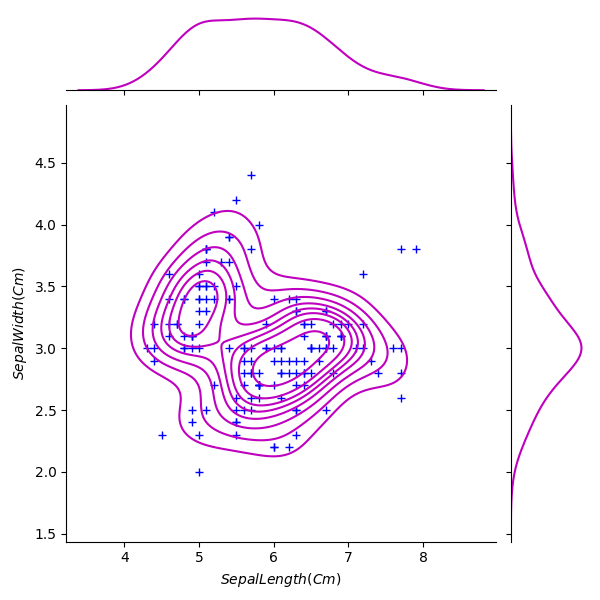﻿ Python Machine learning Scikit-learn: Create a joinplot using “kde” to describe individual distributions on the same plot between Sepal length and Sepal width and use ‘+’ sign as marker - w3resource# Python Scikit-learn: Create a joinplot using “kde” to describe individual distributions on the same plot between Sepal length and Sepal width and use ‘+’ sign as marker

## Python Machine learning Iris Visualization: Exercise-12 with Solution

Write a Python program to create a joinplot using “kde” to describe individual distributions on the same plot between Sepal length and Sepal width and use ‘+’ sign as marker.

Note:
The kernel density estimation (kde) procedure visualize a bivariate distribution. In seaborn, this kind of plot is shown with a contour plot and is available as a style in jointplot().

Sample Solution:

Python Code:

``````import pandas as pd
import seaborn as sns
import matplotlib.pyplot as plt
g = sns.jointplot(x="SepalLengthCm", y="SepalWidthCm", data=iris, kind="kde", color="m")
g.plot_joint(plt.scatter, c="w", s=40, linewidth=1, marker="+")
g.ax_joint.collections.set_alpha(0)
g.set_axis_labels("\$SepalLength(Cm)\$", "\$SepalWidth(Cm)\$")
plt.show()
```
```

Output:

``````

Python Code Editor:

Have another way to solve this solution? Contribute your code (and comments) through Disqus.

Next: Write a Python program to create a pairplot of the iris data set and check which flower species seems to be the most separable.

What is the difficulty level of this exercise?

﻿